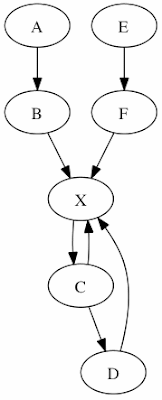### Ranks and snap lines in Instaviz

A Graphviz features is the abilty to align nodes using "ranks" . Does Instaviz  offer a means of using ranks? If not, I imagine a means of dragging a node to "snap" to one of several equidistant lines drawn on the canvas. In this way, the user is able to specify some node organization and still allow Instaviz to automatically layout the graph as a whole. (I think it is reasonable to limit the snap lines to be in one direction only per graph.)

UpdateFor example, I want a graph to have have three ranks: The first rank contains the nodes A, C, and E; The second contains B, D, F; And the third contains X. Without ranks the following definition

`digraph rankexample { A -> B -> X; C -> D -> X; E -> F -> X; C -> X; X -> C;}`

Creates this graph:However, adding ranks (i.e. the "snap lines") to the definition

`digraph rankexample { { rank = same; A; C; E; } { rank = same; B; D; F; } { rank = same; X; } A -> B -> X; C -> D -> X; E -> F -> X; C -> X; X -> C;}`

I get the layout I was looking for: